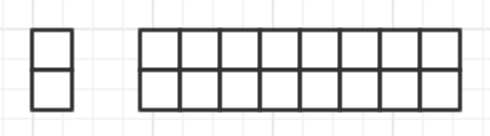### 题目#

1. 编写代码，把字符串中的每个空格替换为`%20`。例如，输入"hello world."，则输出"hello%20world."。
2. 编写代码，给定系数n，求1+2+3+…+n的总和，即`∑`运算符
3. 编写代码，观察如下数列，给定系数n，求数列中的第n个数字(tips: 斐波那契数列)。 `1 1 2 3 5 8 13 21 34 55 89 ...`

### 字符替换#

 `````` 1 2 3 4 5 6 7 8 9 10 11 12 13 14 15 16 17 18 19 20 21 22 23 24 25 26 27 28 29 30 31 32 33 34 35 36 37 38 39 40 41 42 43 44 45 46 47 48 49 50 51 52 53 54 `````` ``````/** * 判断当前字符是否为普通字符 */ public static boolean isPlainChar(char c) { return (c >= '0' && c <= '9') || (c >= 'a' && c <= 'z') || (c >= 'A' && c <= 'Z') || c == '!' || c == '\$' || c == '-' || c == '.' || c == '+' || c == '*' || c == '\'' || c == '(' || c == ')' || c == ','; } public static char[] encodeMap(char c) { //构造特殊字符映射表 String[] map = new String; map[' '] = "%20"; map['/'] = "%2F"; map['?'] = "%3F"; map['%'] = "%25"; map['#'] = "%23"; map['&'] = "%26"; map['='] = "%3D"; return map[c].toCharArray(); } public static char[] urlEncode(char source[]) { int needLength = 0; for (int i = 0; i < source.length; i++) { char c = source[i]; if (isPlainChar(c)) { needLength++; } else { needLength += encodeMap(c).length; } } char result[] = new char[needLength]; int resultIndex = 0; for (int i = 0; i < source.length; i++) { char c = source[i]; if (isPlainChar(c)) { result[resultIndex] = c; resultIndex++; } else { char encodeStr[] = encodeMap(c); System.arraycopy(encodeStr, 0, result, resultIndex, encodeStr.length); resultIndex += encodeStr.length; } } return result; } //=========== //测试代码 public static void main(String args[]) { char[] source = "hello world.".toCharArray(); System.out.println(urlEncode(source)); } ``````

### 累加和 `∑`#

#### 正向循环解题#

 ``````1 2 3 4 5 6 7 `````` ``````public static int sigmaAdd(int n){ int sum = 0; for (int i = 1;i<=n;i++){ sum+=i; } return sum; } ``````

#### 逆向递归求解#

 ``````1 2 3 4 5 6 7 `````` ``````public static int sigmaAdd2(int n) { if (n > 1) { return n + sigmaAdd(--n); } else { return 1; } } ``````

### 斐波那契数列#

#### 递归算法#

1. 当n=0时，f(n) = 0
2. 当0=1时，f(n) = 1
3. 当n>1时，f(n) = f(n-2) + f(n-1)

 `````` 1 2 3 4 5 6 7 8 9 10 11 12 13 14 `````` ``````public static int fibonacci(int n) { if (n <= 0) { return 0; } else if (n < 2) { return 1; } else { return fibonacci(n - 1) + fibonacci(n - 2); } } //=========== //测试代码 public static void main(String args[]){ System.out.println(fibonacci(20)); } ``````

#### 非递归算法#

 `````` 1 2 3 4 5 6 7 8 9 10 11 12 13 `````` ``````public static long fibonacci2(int n) { int fib[] = new int[]{0, 1}; if (n < 2) return fib[n]; long fib1 = 1; long fib2 = 0; long result = 0; for (int i = 2; i <= n; i++) { result = fib1 + fib2; fib2 = fib1; fib1 = result; } return result; } ``````

fib(20)耗时0ms0ms
fib(30)耗时4ms0ms
fib(40)耗时383ms0ms
fib(50)耗时44269ms0ms

### 扩展#

1. 上台阶问题，一共有n个台阶，每次可以上1阶或2阶，那么上到第n阶有几种方法。
2. 小马过河问题，河中有n块石头，小马每次能跳过1块或2块石头，那么跳过n块石头有几种方法。
3. 如下图所示，用左边的2*1的小矩形横着或者竖着去覆盖右边2*8的大矩形，在不发生重叠的情况下，总共有多少种方法？#### 扩展题答案#

• 若上1个台阶，则剩下n-1个台阶
• 若上2个台阶，则剩下n-2个台阶

• 竖着放，则右边还剩2*7的区域，记为f(7)
• 横着放，则左下方也只能横着放一个2*1的小举证，右边还剩下 2*6的区域，记为f(6)